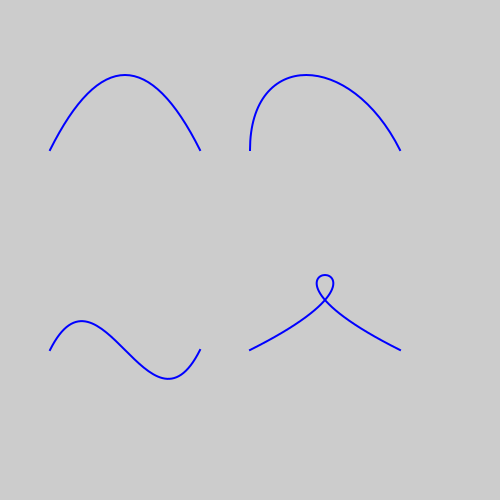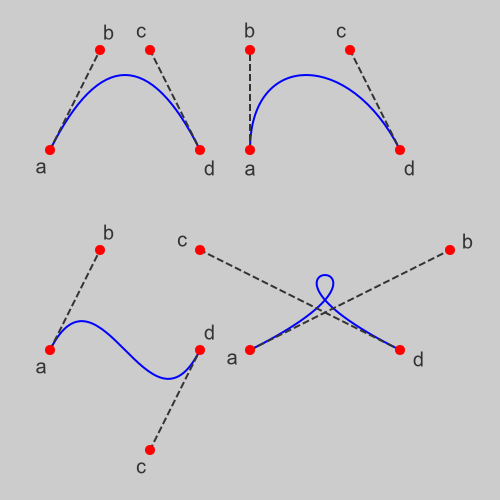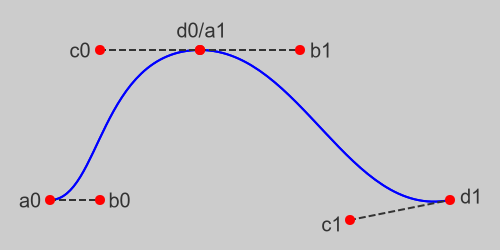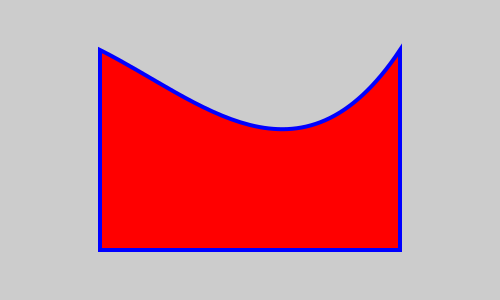# Bezier curves in generativepy

Martin McBride, 2021-12-09
Tags generativepy tutorial bezier curve
Categories generativepy generativepy tutorialThis tutorial shows how to draw Bezier curves in generativepy, using the geometry module.

There are several ways to draw Bezier curves:

• A Bezier object can be used to draw a stand-alone curve.
• Bezier sections can be added to Polygon objects.
• A Bezier object can be included into a complex path, see complex paths.

For simplicity, the shapes are drawn as outlines. See the fill and stroke tutorial for details of how to fill and stroke shapes with different styles, and the patterns tutorial to learn about special fills such as gradients.

## Using Bezier objects

If you are not sure what Bezier curves are, we will describe them a bit more in the next section using generativepy to create some illustrations.

This code draws four simple Bezier curves:

from generativepy.drawing import make_image, setup
from generativepy.color import Color
from generativepy.geometry import Bezier, Polygon, Line, Circle

def draw(ctx, pixel_width, pixel_height, frame_no, frame_count):

setup(ctx, pixel_width, pixel_height, background=Color(0.8))

blue = Color('blue')
thickness = 2

Bezier(ctx).of_abcd((50, 150), (100, 50), (150, 50), (200, 150)).stroke(blue, thickness)
Bezier(ctx).of_abcd((250, 150), (250, 50), (350, 50), (400, 150)).stroke(blue, thickness)
Bezier(ctx).of_abcd((50, 350), (100, 250), (150, 450), (200, 350)).stroke(blue, thickness)
Bezier(ctx).of_abcd((250, 350), (450, 250), (200, 250), (400, 350)).stroke(blue, thickness)

make_image("bezier-tutorial.png", draw, 500, 500)


This code is available on github in tutorial/shapes/bezier.py.

Here is the resulting image:## What are Bezier curves?

A Bezier curve is a versatile type of curve that is often used in vector graphics. It is useful because:

• It can create various types of curves.
• The controls are fairly intuitive.
• Separate curves can be joined to create more complex curved shapes, and it is easy to join curves smoothly if required.

A Bezier curve is defined by 4 control points a, b, c, and d. Here are the curves we drew in the previous section, with the control points marked on each curve:Points a and d are the endpoints of the curve. Point b controls the amount of curvature at the start of the curve. The curve is pulled towards point b. Point c controls the curvature at the and of the curve in a similar way. By moving points b and c we can create U-shapes, S-shapes and even loops.

Here are some other useful facts:

• The entire curve is always contained within the convex hull of the points a, b, c, d.
• The slope of the curve at a is equal to the gradient of the line ab.
• Similarly, the slope of the curve at d is equal to the gradient of the line cd.
• The length of the line ab controls the second derivative (roughly speaking, the "curvature") of the curve at a.
• The length of the line cd controls the second derivative of the curve at d.

It is sometimes useful to create a smooth join between two curves. This allows us to create a complex curved shape that looks as if it was drawn using a single line. If the two curves are described by (a0, b0, c0, d0) and (a1, b1, c1, d1) then to create a smooth join, ensure that:

• Point d0 is equal to point a1. This ensures that the two lines join.
• The line c0d0 should have the same direction as the line a1b1. This ensures that the curves have the same slope where they join.
• The line c0d0 should have the same length as the line a1b1. This ensures that the curves have the same slope where they join.

To create a smooth join, ensure that all 3 conditions are met. This diagram illustrates this:The code to draw these diagrams is in bezier.py on GitHub (see above).

## Adding a Bezier curve to a polygon

It is possible to add Bezier curves in place of one or more sides of a polygon:

def draw3(ctx, pixel_width, pixel_height, frame_no, frame_count):

setup(ctx, pixel_width, pixel_height, background=Color(0.8))

blue = Color('blue')
red = Color('red')
thickness = 4
points = [(100, 250), (100, 50), (200, 100, 300, 200, 400, 50), (400, 250)]
Polygon(ctx).of_points(points).fill(red).stroke(blue, thickness)

make_image("bezier-tutorial-polygon.png", draw3, 500, 300)


Here is the result:In this case:

• The first value in the points list is (100, 250) which sets the start point for the polygon.
• The second value is (100, 50), so a straight line is drawn from the previous point (100, 250) to the new point (100, 50).
• The third value is (200, 100, 300, 200, 400, 50). Since this is a sequence of 6 values rather than 2, it signifies a Bezier segment. The previous point (100, 50) is taken as parameter a of the Bezier. The 6 values in the new parameter represent points b, c and d.
If you found this article useful, you might be interested in the book Computer Graphics in Python or other books by the same author.

#### Popular tags

2d arrays abstract data type alignment and angle animation arange arc array arrays behavioural pattern bezier curve built-in function callable object chain circle classes clipping close closure cmyk colour combinations comparison operator comprehension context context manager conversion count creational pattern data science data types design pattern device space dictionary drawing duck typing efficiency ellipse else encryption enumerate fill filter font font style for loop function function composition function plot functools game development generativepy tutorial generator geometry gif global variable gradient greyscale higher order function hsl html image image processing imagesurface immutable object in operator index inner function input installing iter iterable iterator itertools join l system lambda function len line linear gradient linspace list list comprehension logical operator lru_cache magic method mandelbrot mandelbrot set map matplotlib monad mutability named parameter numeric python numpy object open operator optimisation optional parameter or pandas partial application path pattern permutations polygon positional parameter print pure function python standard library radial gradient range recipes rectangle recursion reduce repeat rgb rotation roundrect scaling scipy sector segment sequence setup shape singleton slice slicing sound spirograph sprite square str stream string stroke structural pattern subpath symmetric encryption template text text metrics tinkerbell fractal transform translation transparency triangle truthy value tuple turtle unpacking user space vectorisation webserver website while loop zip